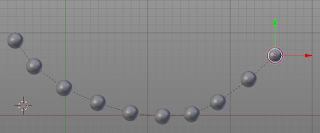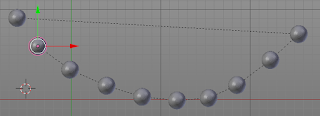### Add-on: Creating a chain of objects, nearest neighbor approximation

In a previous article I started with a very naive solution to create a chain of objects that have a parent-child relation along the shortest path.

This naive solution works but is so slow that even for 10 objects it starts getting unworkable. So the improved version uses a simplistic nearest neighbor approximation that works well with even thousands of objects. It has one drawback, you have to make sure that the active object is at one of the ends of the collection of selected objects because that is where our algorithm starts. This works quite well for artistic use, but in the future I might still try to add the Chistofides algorithm to make it more general.

Anyway, the code is simple enough and the relevant function is shown below:

```def object_list2(objects, active=0):
"""
Return an approximate shortest path through objects starting at the
active index using the nearest neighbor heuristic.
"""

s = time()

# calculate a kd tree to quickly answer nearest neighbor queries
kd = kdtree.KDTree(len(objects))
for i, ob in enumerate(objects):
kd.insert(ob.location, i)
kd.balance()

current = objects[active]
chain = [current]  # we start at the chosen object
for i in range(1,len(objects)):  # we know how many objects to add
# when looking for the nearest neighbor we start with two neigbors
# (because we include the object itself in the search) and if
# the other neigbors is not yet in the chain we add it, otherwise
# we expand our search to a maximum of the total number of objects
for n in range(2,len(objects)):
neighbors = { index for _,index,_ in kd.find_n(current.location, n) }
if neighbors:  # strictly speaking we shoudl assert that len(neighbors) == 1
chain.extend(objects[i] for i in neighbors)
break
current = chain[-1]

print("{n:d} objects {t:.1f}s".format(t=time()-s, n=len(objects)))

return chain```

## Code availability

The full improved version is available on the same GitHub location. (click 'Raw' to download the Python file)

### Add-on: Creating a chain of objects

While working on a project I came across a problem that is surprisingly hard to tackle: chaining a collection of objects along the shortest path.

What I want for this specific example is to parent a collection of objects to each other in such a way that we have a linear chain of parent-child relations. On top of that I want this chain to be as short as possible, that is, going from parent to child along the chain of objects, I want the length of this path to be minimal.

To illustrate what I mean, the first image shows what I am after while the second image shows a decidedly sub-optimal chain of parent-child relations:The problem itself is well known (finding the shortest Hamiltonian path, closely related to the Traveling Salesman problem) but unfortunately solving this problem exactly is very costly in computational terms.

The code below shows a working but very naive implementation that I intend to use as a starting point for later improvements. It works in the sense that it finds the shortest path between a collection of selected objects and creates the chain of parent-child relations but the time to compute the solution increases more that exponentially (factorial to be precise: 8 objects will for example take 0.1 seconds, 9 objects will take nine times as much, i.e. almost 1 second and 10 objects will take ten times as much still, i.e. 10 seconds and so on). To make this remotely useful, for example to chain a necklace of 100 beads, we will have to implement some clever heuristics. That is something I intend to cover in future articles.

## Code availability

The current code is shown in full below but the add-on as it evolves will be available on GitHub. (click 'Raw' to download the Python file)
```import bpy
from mathutils import kdtree
from itertools import permutations as perm
from functools import lru_cache
from time import time
from math import factorial as fac

bl_info = {
"name": "Chain selected objects",
"author": "Michel Anders (varkenvarken)",
"version": (0, 0, 201701220957),
"blender": (2, 78, 0),
"location": "View3D > Object > Chain selected objects",
"description": """Combine selected objects to a list of parent-child relations based on proximity""",
"category": "Object"}

def object_list(objects):
"""
Return the shortest Hamiltonian path through a collection of objects.

This is calculated using a brute force method that is certainly not
intented for real life use because for example going from ten to
eleven objects will increase the running time elevenfold and even
with caching expensive distance calculations this quickly becomes
completely unworkable.

But this routine is intended as our baseline algorithm that is meant
to be replaced with an approximation algorithm that is 'good enough'
for our purposes.
"""
@lru_cache()
def distance_squared(a,b):
return (objects[a].location-objects[b].location).length_squared

def length_squared(chain):
sum = 0.0
for i in range(len(chain)-1):
sum += distance_squared(chain[i],chain[i+1])
return sum

s = time()

shortest_d2 = 1e30
shortest_chain = None

n_half = fac(len(objects))//2
for i,chain in enumerate(perm(range(len(objects)))):
if i >= n_half:
break
d2 = length_squared(chain)
if d2 < shortest_d2:
shortest_d2 = d2
shortest_chain = chain

print("{n:d} objects {t:.1f}s".format(t=time()-s, n=len(objects)))

return [objects[i] for i in shortest_chain]

class ChainSelectedObjects(bpy.types.Operator):
bl_idname = 'object.chainselectedobjects'
bl_label = 'Chain selected objects'
bl_options = {'REGISTER', 'UNDO'}

@classmethod
def poll(self, context):
return (context.mode == 'OBJECT'
and len(context.selected_objects) > 1)

def execute(self, context):
objects = object_list(context.selected_objects.copy())
for ob in objects:
ob.select = False

ob = objects.pop()
first = ob
while objects:
context.scene.objects.active = ob
child = objects.pop()
child.select = True
bpy.ops.object.parent_set(keep_transform=True)
child.select = False
ob = child
first.select = True
context.scene.objects.active = first
return {"FINISHED"}

self.layout.operator(
ChainSelectedObjects.bl_idname,
text=ChainSelectedObjects.bl_label,
icon='PLUGIN')

def register():
bpy.utils.register_module(__name__)